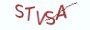Python基础教程

# 14.4.1 理解匿名函数# 14.4.2 lambda表达式

>>> help("lambda")
Lambdas
*******
lambda_expr        ::= "lambda" [parameter_list] ":" expression
lambda_expr_nocond ::= "lambda" [parameter_list] ":" expression_nocond
Lambda expressions (sometimes called lambda forms) are used to create
anonymous functions. The expression "lambda parameters: expression"
yields a function object.  The unnamed object behaves like a function
object defined with:
def <lambda>(parameters):
return expression
See section Function definitions for the syntax of parameter lists.
Note that functions created with lambda expressions cannot contain
statements or annotations.
Related help topics: FUNCTIONS

Lambda expressions (sometimes called lambda forms) are used to create anonymous functions.
lambda表达式的语法结构：
lambda parameters: expression
parameters表示函数的形参列表，参数以逗号进行分隔，形参列表与具名函数一样，可以为空。expression表示你要实现的表达式的代码逻辑，表达式的值会直接返回给函数调用方，无需通过return进行返回。

```# __desc__ = 使用具名函数和匿名函数来编写一段相同逻辑的代码

# 1. 定义具名函数accumulate，将参数进行累加
def accumulate(small, large):
return small + large

x = 1
y = 2

z = accumulate(x, y)
# z的输出为3

# 2. 定义匿名函数，将参数进行累加
# 由于是匿名函数，所以不能直接调用该匿名函数，需要将匿名函数保存到一个变量中，
# 再进行调用

anonymous_accumulate = lambda x, y:x+y

# 通过变量来调用匿名函数时，与具名函数的调用方式一样
z = anonymous_accumulate(x, y)
# z的输出为3```

# 14.4.3  lambda表达式与具名函数

lambda表达式与具名函数相比，在形式上更加简单，只需一行代码就能搞定。但也同时限制了lambda表达式不能实现复杂的代码逻辑。如要实现复杂的代码逻辑，还应当使用具名函数。lambda表达式使用起来很灵活，可以作为参数传递，作为返回值来传回给调用方，也可以直接嵌入到其它数据结构中。
(1) 使用lambda表达式作为参数的默认值

```# __desc__ = 使用lambda表达式作为默认参数

# 1. 定义具名函数accumulate，将参数进行累加
return small + large

x = 1
y = 1
z = accumulate(1,2)
# z的输出为4```

```# __desc__ = 使用lambda表达式作为默认参数

# 1. 定义具名函数accumulate，将参数进行累加
return small + large

# 使用def定义一个加权函数
return x+1

x = 1
y = 1

# z的输出同样为4```
(2) 将lambda表达式嵌入到字典结构中

```# __desc__ = 将lambda表达式嵌入到字典结构中

# 定义一个commands字典，表示各种命令
commands = {"add": lambda x,y:x+y, "sub": lambda x, y:x-y}

x = 1
y = 2

# 1.执行加法运算
# z的输出为3

# 2.执行减法运算
z = commands["sub"](x,y)
# z的输出为-1```
(3) 将lambda表达式作为返回值

```# __desc__ = 将lambda表达式作为值进行返回

# 在具名函数中返回lambda表达式
def get_accumulate_lambda(x,y):
initial_value = 2020
return lambda :x+y+initial_value

accumulate = get_accumulate_lambda(0,1)
z = accumulate()
# z的输出为2021```

```# __desc__ = 将lambda表达式作为值进行返回

# 在具名函数中返回lambda表达式
def get_accumulate(x,y):
# 在函数中定义函数
def accumulate():
initial_value = 2020
return x+y+initial_value

# 定义的函数作为返回值进行返回
return accumulate

accumulate = get_accumulate(0,1)
z = accumulate()
# z的输出为2021```

# 14.4.4 知识要点

(1) 匿名函数，即不具名，没有函数名的函数。
(2) 在Python中通过lambda表达式来定义匿名函数。使用匿名函数时，要先通过变量来保存匿名函数的地址。这样就可以在下文中进行调用。
(3) lambda表达式与具名函数相比，在形式上更加简单，只需一行代码。

# 14.4.5 最具实力的小班培训

`(1) Python后端工程师高薪就业班，月薪11K-18K，免费领取课程大纲(2) Python爬虫工程师高薪就业班，年薪十五万，免费领取课程大纲(3) Java后端开发工程师高薪就业班，月薪11K-20K, 免费领取课程大纲(4) Python大数据分析，量化投资就业班，月薪12K-25K,免费领取课程大纲`## 注册账号